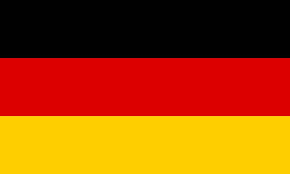# Dot productEnter two vectors. Mathepower calculates their dot product.

 u =and v=## How to calculate the dot product?

It is quite simple: Just multiply the vectors line by line and add the results.

## And why do that?

Because the dot product has many useful applications. It can be used to compute the angle between vectors. And whenever the vectors are perpendicular to each other, the dot product is equal to 0.

## How can I calculate the dot product?

Just enter it above…